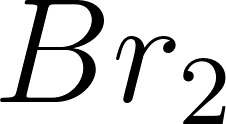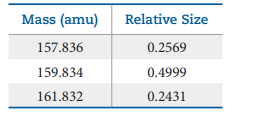×
Get Full Access to Chemistry: The Central Science - 13 Edition - Chapter 22 - Problem 4e
Get Full Access to Chemistry: The Central Science - 13 Edition - Chapter 22 - Problem 4e

×

# ?4EVisualizing ConceptsYou have two glass bottles, oneISBN: 9780321910417 77

## Solution for problem 4E Chapter 22

Chemistry: The Central Science | 13th Edition

• Textbook Solutions
• 2901 Step-by-step solutions solved by professors and subject experts
• Get 24/7 help from StudySoup virtual teaching assistantsChemistry: The Central Science | 13th Edition

4 5 1 315 Reviews
21
4
Problem 4E

These exercises are not divided by category, although they are roughly in the order of the topics in the chapter. They are not paired.

There are two different isotopes of bromine atoms. Under normal conditions, elemental bromine consists ofmolecules, and the mass of amolecule is the sum of the masses of the two atoms in the molecule. The mass spectrum ofconsists of three peaks:(a) What is the origin of each peak (of what isotopes does each consist)? (b) What is the mass of each isotope? (c) Determine the average molecular mass of amolecule, (d) Determine the average atomic mass of a bromine atom, (e) Calculate the abundances of the two isotopes.

Step-by-Step Solution:

Step 1 of 6

Chemical elements with the same number of protons but differing quantities of neutrons are called isotopes.Isotopes are distinguished by their mass, which is equal to the sum of their protons and neutrons.

Step 2 of 6

Step 3 of 6

##### ISBN: 9780321910417

This full solution covers the following key subjects: . This expansive textbook survival guide covers 305 chapters, and 6352 solutions. Chemistry: The Central Science was written by and is associated to the ISBN: 9780321910417. Since the solution to 4E from 22 chapter was answered, more than 265 students have viewed the full step-by-step answer. The answer to “?These exercises are not divided by category, although they are roughly in the order of the topics in the chapter. They are not paired.There are two different isotopes of bromine atoms. Under normal conditions, elemental bromine consists of molecules, and the mass of a molecule is the sum of the masses of the two atoms in the molecule. The mass spectrum of consists of three peaks: (a) What is the origin of each peak (of what isotopes does each consist)? (b) What is the mass of each isotope? (c) Determine the average molecular mass of a molecule, (d) Determine the average atomic mass of a bromine atom, (e) Calculate the abundances of the two isotopes.” is broken down into a number of easy to follow steps, and 115 words. The full step-by-step solution to problem: 4E from chapter: 22 was answered by , our top Chemistry solution expert on 09/04/17, 09:30PM. This textbook survival guide was created for the textbook: Chemistry: The Central Science, edition: 13.

Unlock Textbook Solution